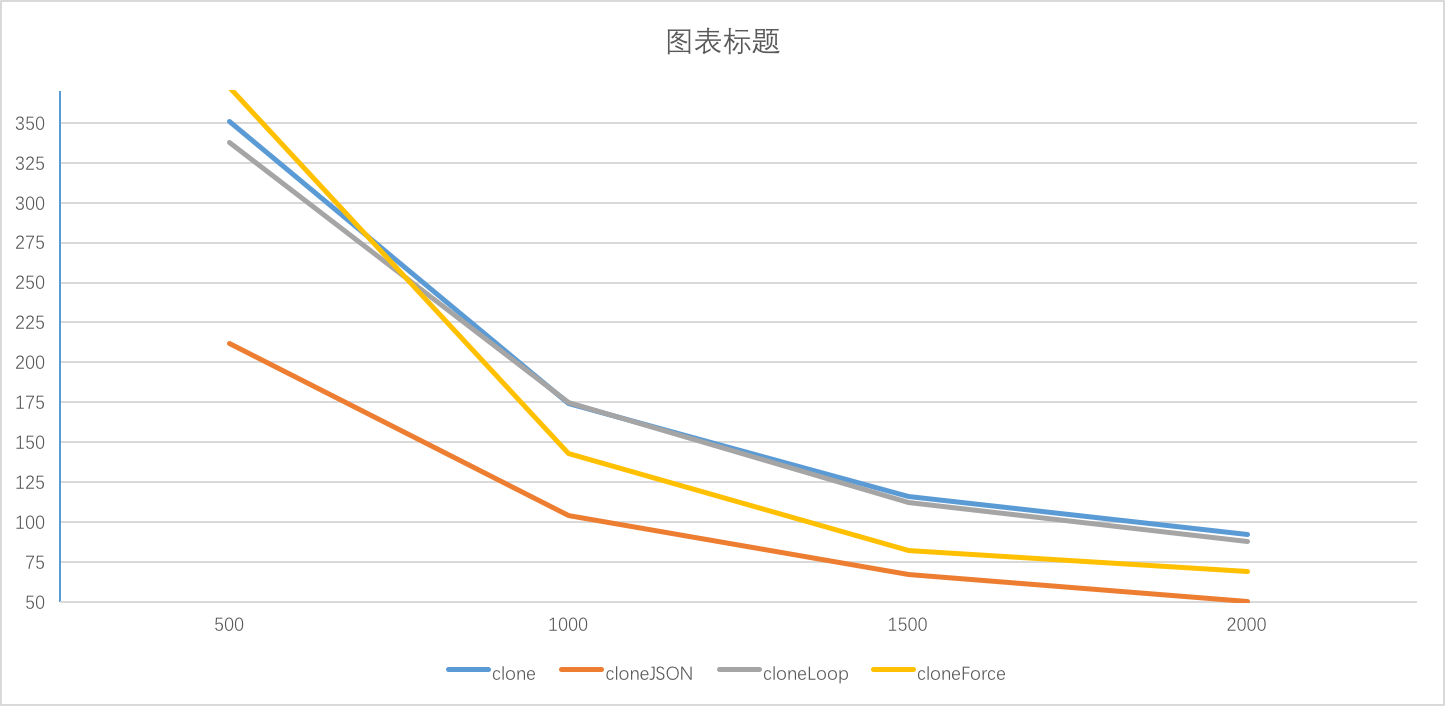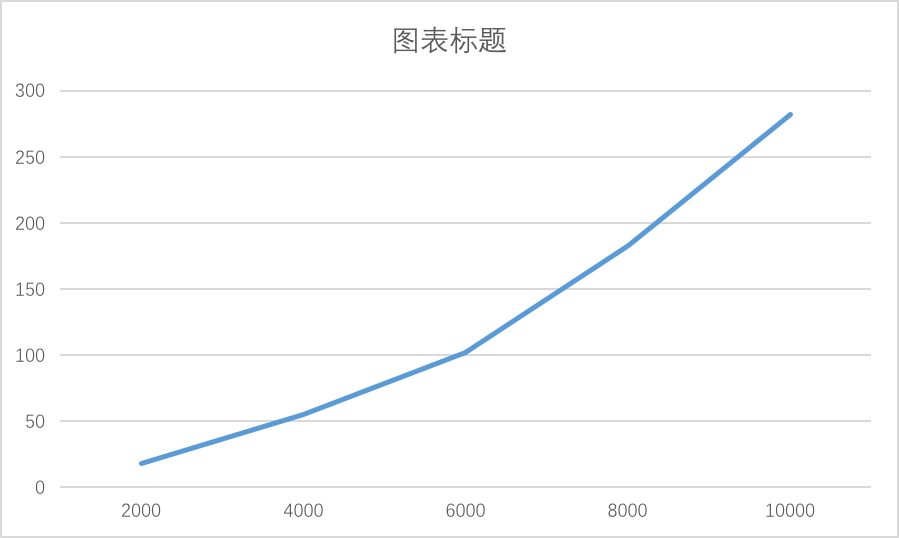10 October 2018

## 深拷贝 VS 浅拷贝

``````// 基本类型
var a = 1;
var b = a;
a = 2;
console.log(a, b); // 2, 1 ，a b指向不同的数据

// 引用类型指向同一份数据
var a = {c: 1};
var b = a;
a.c = 2;
console.log(a.c, b.c); // 2, 2 全是2，a b指向同一份数据
``````

``````var a1 = {b: {c: {}};

var a2 = shallowClone(a1); // 浅拷贝
a2.b.c === a1.b.c // true

var a3 = clone(a1); // 深拷贝
a3.b.c === a1.b.c // false
``````

``````function shallowClone(source) {
var target = {};
for(var i in source) {
if (source.hasOwnProperty(i)) {
target[i] = source[i];
}
}

return target;
}
``````

## 最简单的深拷贝

``````var a1 = {b: {c: {d: 1}};
``````

``````function clone(source) {
var target = {};
for(var i in source) {
if (source.hasOwnProperty(i)) {
if (typeof source[i] === 'object') {
target[i] = clone(source[i]); // 注意这里
} else {
target[i] = source[i];
}
}
}

return target;
}
``````

• 没有对参数做检验
• 判断是否对象的逻辑不够严谨
• 没有考虑数组的兼容

(⊙o⊙)，下面我们来看看各个问题的解决办法，首先我们需要抽象一个判断对象的方法，其实比较常用的判断对象的方法如下，其实下面的方法也有问题，但如果能够回答上来那就非常不错了，如果完美的解决办法感兴趣，不妨看看这里吧

``````function isObject(x) {
return Object.prototype.toString.call(x) === '[object Object]';
}
``````

``````function clone(source) {
if (!isObject(source)) return source;

// xxx
}
``````

``````function createData(deep, breadth) {
var data = {};
var temp = data;

for (var i = 0; i < deep; i++) {
temp = temp['data'] = {};
for (var j = 0; j < breadth; j++) {
temp[j] = j;
}
}

return data;
}

createData(1, 3); // 1层深度，每层有3个数据 {data: {0: 0, 1: 1, 2: 2}}
createData(3, 0); // 3层深度，每层有0个数据 {data: {data: {data: {}}}}
``````

``````clone(createData(1000)); // ok
clone(createData(10000)); // Maximum call stack size exceeded

clone(createData(10, 100000)); // ok 广度不会溢出
``````

``````var a = {};
a.a = a;

clone(a) // Maximum call stack size exceeded 直接死循环了有没有，/(ㄒoㄒ)/~~
``````

## 一行代码的深拷贝

``````function cloneJSON(source) {
return JSON.parse(JSON.stringify(source));
}
``````

``````cloneJSON(createData(10000)); // Maximum call stack size exceeded
``````

``````var a = {};
a.a = a;

cloneJSON(a) // Uncaught TypeError: Converting circular structure to JSON
``````

## 破解递归爆栈

``````var a = {
a1: 1,
a2: {
b1: 1,
b2: {
c1: 1
}
}
}
``````

``````    a
/   \
a1   a2
|    / \
1   b1 b2
|   |
1  c1
|
1
``````

``````function cloneLoop(x) {
const root = {};

// 栈
const loopList = [
{
parent: root,
key: undefined,
data: x,
}
];

while(loopList.length) {
// 深度优先
const node = loopList.pop();
const parent = node.parent;
const key = node.key;
const data = node.data;

// 初始化赋值目标，key为undefined则拷贝到父元素，否则拷贝到子元素
let res = parent;
if (typeof key !== 'undefined') {
res = parent[key] = {};
}

for(let k in data) {
if (data.hasOwnProperty(k)) {
if (typeof data[k] === 'object') {
// 下一次循环
loopList.push({
parent: res,
key: k,
data: data[k],
});
} else {
res[k] = data[k];
}
}
}
}

return root;
}
``````

## 破解循环引用

``````var b = {};
var a = {a1: b, a2: b};

a.a1 === a.a2 // true

var c = clone(a);
c.a1 === c.a2 // false
``````

`find`是抽象的一个函数，其实就是遍历`uniqueList`

``````// 保持引用关系
function cloneForce(x) {
// =============
const uniqueList = []; // 用来去重
// =============

let root = {};

// 循环数组
const loopList = [
{
parent: root,
key: undefined,
data: x,
}
];

while(loopList.length) {
// 深度优先
const node = loopList.pop();
const parent = node.parent;
const key = node.key;
const data = node.data;

// 初始化赋值目标，key为undefined则拷贝到父元素，否则拷贝到子元素
let res = parent;
if (typeof key !== 'undefined') {
res = parent[key] = {};
}

// =============
// 数据已经存在
let uniqueData = find(uniqueList, data);
if (uniqueData) {
parent[key] = uniqueData.target;
continue; // 中断本次循环
}

// 数据不存在
// 保存源数据，在拷贝数据中对应的引用
uniqueList.push({
source: data,
target: res,
});
// =============

for(let k in data) {
if (data.hasOwnProperty(k)) {
if (typeof data[k] === 'object') {
// 下一次循环
loopList.push({
parent: res,
key: k,
data: data[k],
});
} else {
res[k] = data[k];
}
}
}
}

return root;
}

function find(arr, item) {
for(let i = 0; i < arr.length; i++) {
if (arr[i].source === item) {
return arr[i];
}
}

return null;
}
``````

``````var b = {};
var a = {a1: b, a2: b};

a.a1 === a.a2 // true

var c = cloneForce(a);
c.a1 === c.a2 // true
``````

``````var a = {};
a.a = a;

cloneForce(a)
``````

## 性能对比

``````function runTime(fn, time) {
var stime = Date.now();
var count = 0;
while(Date.now() - stime < time) {
fn();
count++;
}

return count;
}

runTime(function () { clone(createData(500, 1)) }, 2000);
``````

500 351 212 338 372
1000 174 104 175 143
1500 116 67 112 82
2000 92 50 88 69

• 随着深度变小，相互之间的差异在变小
• clone和cloneLoop的差别并不大
• cloneLoop > cloneForce > cloneJSON• clone时间 = 创建递归函数 + 每个对象处理时间
• cloneJSON时间 = 循环检测 + 每个对象处理时间 * 2 （递归转字符串 + 递归解析）
• cloneLoop时间 = 每个对象处理时间
• cloneForce时间 = 判断对象是否缓存中 + 每个对象处理时间

cloneJSON的速度只有clone的50%，很容易理解，因为其会多进行一次递归时间

cloneForce由于要判断对象是否在缓存中，而导致速度变慢，我们来计算下判断逻辑的时间复杂度，假设对象的个数是n，则其时间复杂度为O(n2)，对象的个数越多，cloneForce的速度会越慢

``````1 + 2 + 3 ... + n = n^2/2 - 1
``````

0 13400 3272 14292 989

• 随着对象的增多，cloneForce的性能低下凸显
• cloneJSON的性能也大打折扣，这是因为循环检测占用了很多时间
• cloneLoop的性能高于clone，可以看出递归新建函数的时间和循环对象比起来可以忽略不计

``````var data1 = createData(2000, 0);
var data2 = createData(4000, 0);
var data3 = createData(6000, 0);
var data4 = createData(8000, 0);
var data5 = createData(10000, 0);

cloneForce(data1)
cloneForce(data2)
cloneForce(data3)
cloneForce(data4)
cloneForce(data5)
``````## 总结

clone cloneJSON cloneLoop cloneForce

``````// npm install --save @jsmini/clone
import { clone, cloneJSON, cloneLoop, cloneForce } from '@jsmini/clone';
``````

@jsmini/clone孵化于jsmini，jsmini致力于为大家提供一组小而美，无依赖的高质量库

jsmini的诞生离不开jslib-base，感谢jslib-base为jsmini提供了底层技术